# 0. 引言

• 注：后文中所有的表示未标注的话均为向量表示法

# 1. 法一（基本法曰.. 曰）

## 定义

• 一条直线由一个点 $O$和一个向量 $V$组成，过点 $O,P$的直线可以表示为：$O+kV$，其中 $V=\overrightarrow{OP}$。
• $O=(x,y),P=(x1,y1),\overrightarrow{OP}=(x1-x,y1-y)$
• 一条线段由两个点构成

## 1. 判断线段与直线相交

### 正文

• 1.$\overrightarrow{p0p2}$在 $\overrightarrow{p0p1}$逆时针方向 (Counter Clock Wise)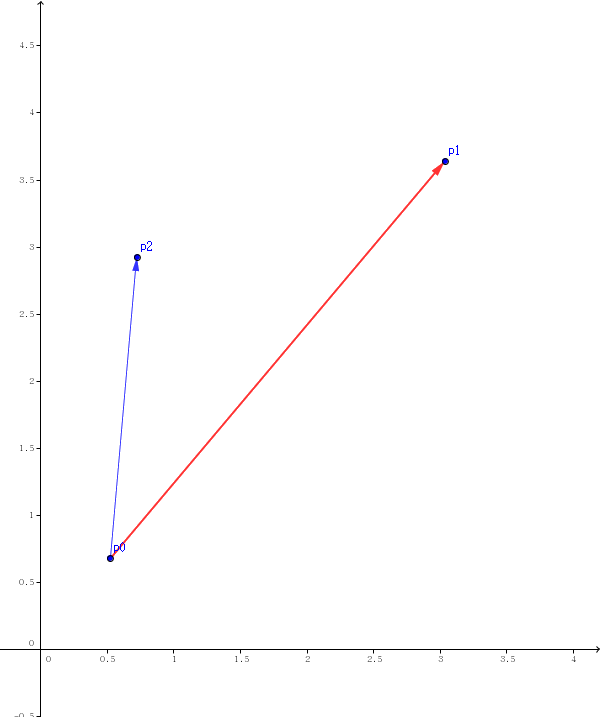• 2.$\overrightarrow{p0p2}$在 $\overrightarrow{p0p1}$顺时针方向 (Clock Wise)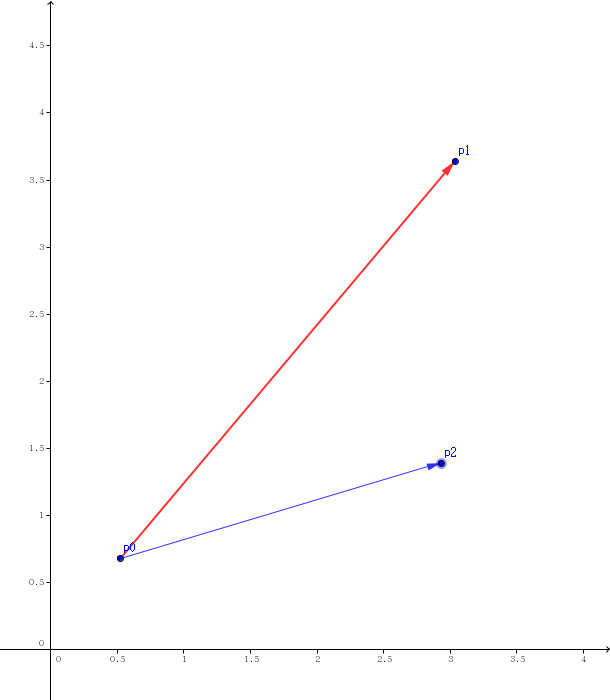• 3.$p2$在直线 $p0p1$上且 $\overrightarrow{p0p1}$与 $\overrightarrow{p0p2}$反向 (On Line Back)• 4.$p2$在直线 $p0p1$上且 $\overrightarrow{p0p1}$与 $\overrightarrow{p0p2}$同向且 $p2$不在线段 $p0p1$上 (On Line Front)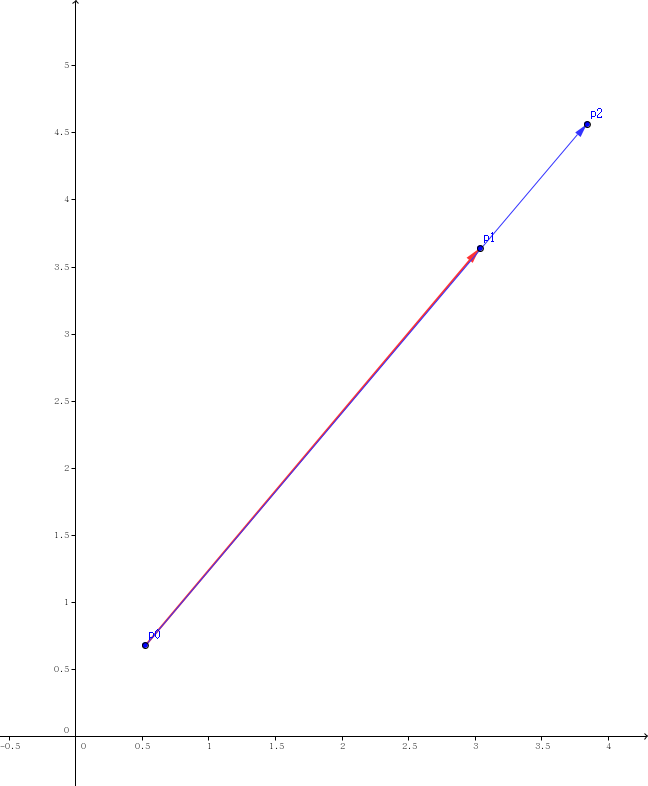• 5.$p2$在直线 $p0p1$上且 $\overrightarrow{p0p1}$与 $\overrightarrow{p0p2}$同向且 $p2$在线段 $p0p1$上 (On segment)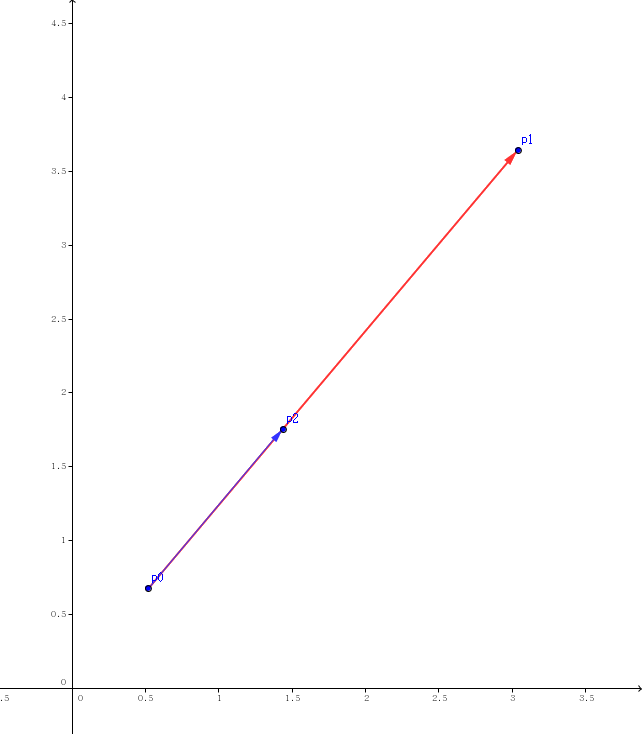• $p0,p1$分别在 $\overrightarrow{p2p3}$两端，一个在顺时针方向，一个在逆时针方向。
• $p0,p1$中至少有 1 个在线段 $p2p3$上
• $p0,p1,p2,p3$在同一直线上，且线段 $p2,p3$在线段 $p0,p1$上

#define EPS (1e-10)
typedef struct Point//点与向量的结构体
{
double x,y;
double dot(Point a){return x*a.x+y*a.y;}//点乘
double cross(Point a){return x*a.y-a.x*y;}//叉乘
double norm(){return x*x+y*y;}//计算向量大小
Point operator - (Point a){return (Point){x-a.x,y-a.y};}//向量相减
}Vector;
double ccw(Point s,Point e,Point p)//Counter Clock wise, 判断点与线段的位置关系
{
//s 是线段起点，e 是终点，p 是需要判断的点
Vector a=e-s,b=p-s;//那么这里的 a 就上面五张图中红色的向量，b 是蓝色的向量
if(a.cross(b)>EPS)return 1;//若 a 与 b 的叉积为正就说明是情况 1，即点在线段逆时针方向
if(a.cross(b)<-EPS)return -1;//若 a 与 b 的叉积为负就说明是情况 2，即点在线段顺时针方向
//剩下三种情况为点在线段所在直线上
if(a.dot(b)<-EPS)return 2;//若 a 与 b 的点积为负，则说明两向量反向，为情况 3
if(a.norm()<b.norm())return -2;//若 a 的大小小于 b 的大小，说明是情况 4
return 0;//点在线段上，情况 5
}


1. $p0,p1$分别在 $\overrightarrow{p2p3}$两端，一个在顺时针方向，一个在逆时针方向。此时 $ccw(p2,p3,p0)\times ccw(p2,p3,p1)=-1$
2. $p0,p1$中至少有 1 个在线段 $p2p3$上，此时 $ccw(p2,p3,p0)\times ccw(p2,p3,p1)=0$
3. $p0,p1,p2,p3$在同一直线上，且线段 $p2,p3$在线段 $p0,p1$上，此时 $ccw(p2,p3,p0)\times ccw(p2,p3,p1)=-4$

bool intersect(Point p0,Point p1,Point p2,Point p3)
{
return (ccw(p0,p1,p2)*ccw(p0,p1,p3)<=0&&ccw(p2,p3,p0)*ccw(p2,p3,p1)<=0);
}


### 例题

You can Solve a Geometry Problem too
HDU – 1086: 传送门=￣ω￣=

#include <bits/stdc++.h>
#define EPS (1e-10)
using namespace std;
typedef struct Point
{
double x,y;
double dot(Point a){return x*a.x+y*a.y;}
double cross(Point a){return x*a.y-a.x*y;}
double norm(){return x*x+y*y;}
Point operator - (Point a){return (Point){x-a.x,y-a.y};}
}Vector;
int n,ans;
Point ps,pe;
double ccw(Point s,Point e,Point p)
{
Vector a=e-s,b=p-s;
if(a.cross(b)>EPS)return 1;
if(a.cross(b)<-EPS)return -1;
if(a.dot(b)<-EPS)return 2;
if(a.norm()<b.norm())return -2;
return 0;
}
bool intersect(Point p0,Point p1,Point p2,Point p3)
{
return (ccw(p0,p1,p2)*ccw(p0,p1,p3)<=0&&ccw(p2,p3,p0)*ccw(p2,p3,p1)<=0);
}
int main()
{
while(scanf("%d",&n),ans=0,n)
{
for(int i=1;i<=n;i++)
scanf("%lf%lf%lf%lf",&ps[i].x,&ps[i].y,&pe[i].x,&pe[i].y);
for(int i=1;i<=n;i++)
for(int j=i+1;j<=n;j++)
ans+=intersect(ps[i],pe[i],ps[j],pe[j]);
printf("%d\n",ans);
}
return 0;
}


## 2. 判断线段与直线相交

### 正文

int ccw(Point s,Vector v,Point p)//判断直线 s+kv 与点 p 的关系
{
Vector v2=p-s;
if(v.cross(v2)>EPS)return 1;//点在直线逆时针方向
if(v.cross(v2)<-EPS)return -1;//点在直线顺时针方向
return 0;//点在直线上
}


bool intersect(Point s,Vector v,Point p1,Point p2)
{
return (ccw(s,v,p1)*ccw(s,v,p2)<=0);
}


### 例题

Segments
POJ – 3304: 传送门=￣ω￣=

（我之前把特判写读入前面了WA了好久！

#include <cstdio>
#include <cmath>
#define NS (105)
#define EPS (1e-10)
using namespace std;
typedef struct Point
{
double x,y;
double cross(Point a){return x*a.y-a.x*y;}
Point operator - (Point a)const{return (Point){x-a.x,y-a.y};}
bool operator != (Point a)const{return fabs(x-a.x)>EPS||fabs(y-a.y)>EPS;}
}Vector;
int T,n;
Point p[NS];
int ccw(Point s,Vector v,Point p)
{
Vector v2=p-s;
if(v.cross(v2)>EPS)return 1;
if(v.cross(v2)<-EPS)return -1;
return 0;
}
bool intersect(Point s,Vector v,Point p1,Point p2)
{
return (ccw(s,v,p1)*ccw(s,v,p2)<=0);
}
int main()
{
scanf("%d",&T);
while(T--)
{
scanf("%d",&n);
for(int i=1;i<=n;i++)
scanf("%lf%lf%lf%lf",&p[i].x,&p[i].y,&p[i].x,&p[i].y);
if(n==1){puts("Yes!");goto nxt;}
Vector v;
for(int i=1;i<=n;i++)
for(int j=i+1;j<=n;j++)
for(int x0=0;x0<=1;x0++)
for(int x1=0;x1<=1;x1++)
if(p[x0][i]!=p[x1][j])
{
v=p[x1][j]-p[x0][i];
for(int k=1;k<=n;k++)
if(!intersect(p[x0][i],v,p[k],p[k]))goto end;
puts("Yes!");goto nxt;
end:continue;
}
puts("No!");
nxt:continue;
}
return 0;
}


# 法二 (二次判断法)

• 如果包裹两条线段的矩形没有相交，那么两条线段一定没有相交，反之不一定。(排斥实验)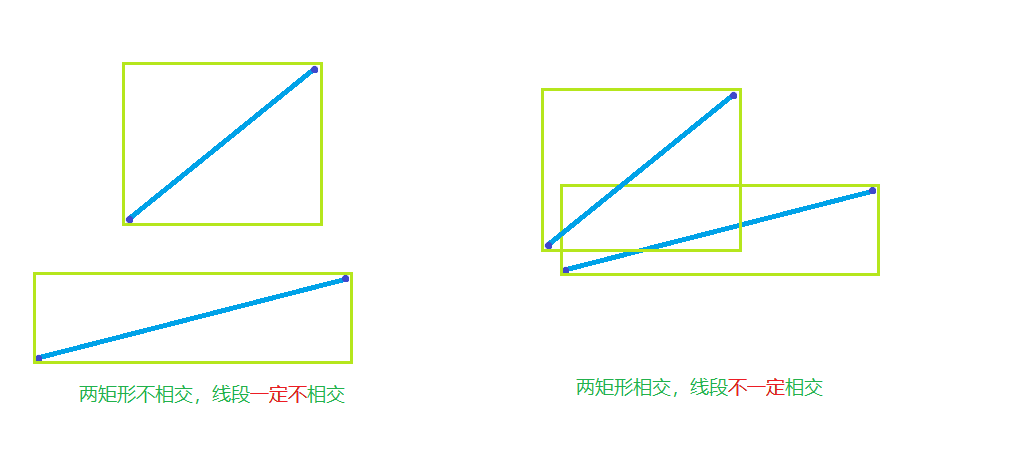• 如果线段彼此跨过对方所在的直线或端点在直线上，则两线段一定相交。(跨立实验)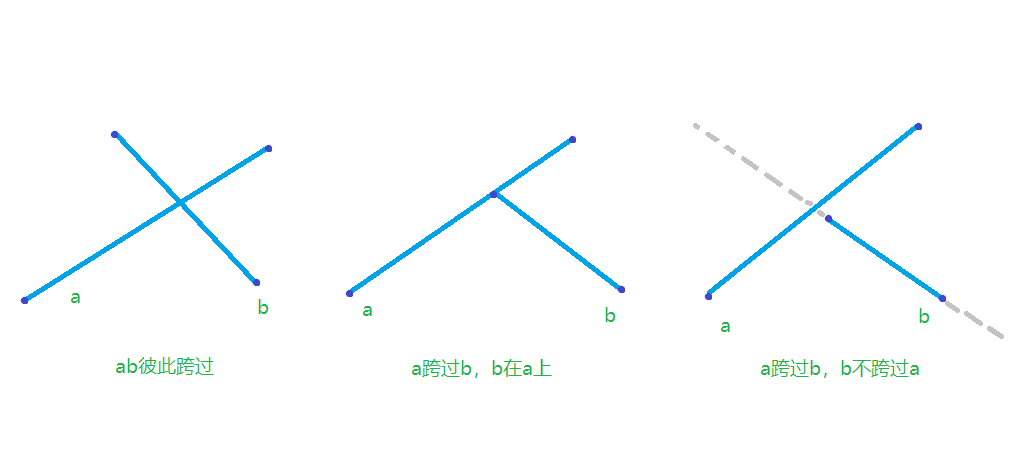typedef struct tvec
{
double x,y;
tvec operator - (const tvec a)const{return (tvec){x-a.x,y-a.y};}
}vec;
double crs(vec a,vec b){return a.x*b.y-a.y*b.x;}

bool Intersect(vec s1,vec e1,vec s2,vec e2)
{
if( min(s1.y,e1.y)>max(s2.y,e2.y)||                     //排斥实验
max(s1.y,e1.y)<min(s2.y,e2.y)||
min(s1.x,e1.x)>max(s2.x,e2.x)||
max(s1.x,e1.x)<min(s2.x,e2.x))return 0;
if( crs(s1-e2,s2-e2)*crs(e1-e2,s2-e2)<=0&&              //跨立实验
crs(e2-s1,e1-s1)*crs(s2-s1,e1-s1)<=0)return 1;
else return 0;
}


### 4 条评论#### litble · 2018年1月19日 8:24 下午

point jd(point a1,point b1,point a2,point b2) {
db t1=(b1-a1)*(a1-a2),t2=(b1-a1)*(b2-a2),t=t1/t2;
return (point){a2.x+t*(b2.x-a2.x),a2.y+t*(b2.y-a2.y)};
}
int jiao(point as,point bs) {
if((as.x-bs.x)*(as.x-bs.x)+(as.y-bs.y)*(as.y-bs.y)<eps) return 0;
for(int i=1;i<=n;++i) {
if(fabs((l[i].b-l[i].a)*(bs-as))<eps)//特判平行
if(fabs((bs-as)*(l[i].b-as))<eps) continue;
else return 0;
point o=jd(as,bs,l[i].a,l[i].b);//求交点
if(l[i].a.x>o.x+eps||o.x>l[i].b.x+eps) return 0;//看是否在线段上
if(min(l[i].a.y,l[i].b.y)>o.y+eps||max(l[i].a.y,l[i].b.y)+eps<o.y) return 0;
}
return 1;
}